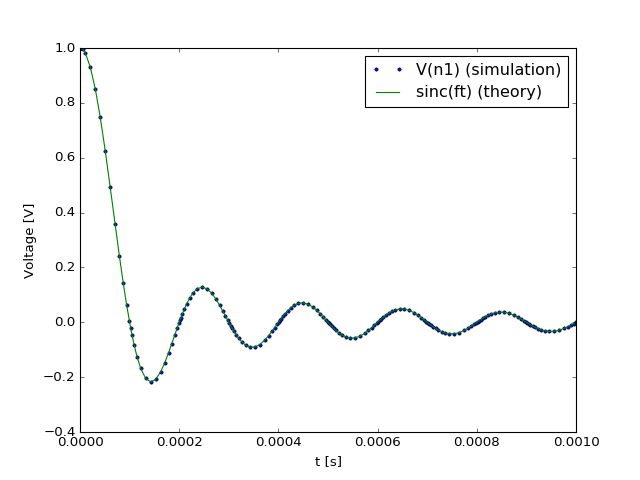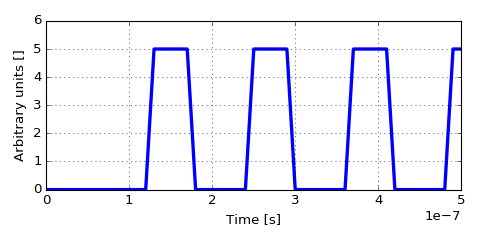# ahkab.time_functions¶

This module contains several basic time functions.

The classes that are found in module are useful to provide a time-varying characteristic to independent sources.

Notice that the time functions are not restricted to those provided here, the user is welcome to provide his own. Implementing a custom time function is easy and common practice, as long as you are interfacing to the simulator through Python. Please see the dedicated section Defining custom time functions below.

## Classes defined in this module¶

 pulse(v1, v2, td, tr, pw, tf, per) Square wave aka pulse function pwl(x, y[, repeat, repeat_time, td]) Piece-Wise Linear (PWL) waveform sin(vo, va, freq[, td, theta, phi]) Sine wave exp(v1, v2, td1, tau1, td2, tau2) Exponential wave sffm(vo, va, fc, mdi, fs, td) Single-Frequency FM (SFFM) waveform am(sa, fc, fm, oc, td) Amplitude Modulated (AM) waveform

## Supplying a time function to an independent source¶

Providing a time-dependent characteristic to an independent source is very simple and probably best explained with an example.

Let’s say we wish to define a sinusoidal voltage source with no offset, amplitude 5V and 1kHz frequency.

It is done in two steps:

• first we define the time function with the built-in class ahkab.time_functions.sin:

sin1k = time_functions.sin(vo=0, va=5, freq=1e3)

• Then we define the voltage source and we assign the time function to it:

cir.add_vsource('V1', 'n1', cir.gnd, 1, function=mys)


In the example above, the sine wave is assigned to a voltage source 'V1', that gets added to a circuit cir (not shown).

## Defining custom time functions¶

Defining a custom time function is easy, all you need is either:

• A function that takes a float (the time) and returns the function value,
• An instance with a __call__(self, time) method. This solution allows having internal parameters, typically set through the constructor.

In both cases, in time-based simulations, the simulator will call the object at every time step, supplying a single parameter, the simulation time (time in the following, of type float).

In turn, the simulator expects to receive as return value a float, corresponding to the value of the time-dependent function at the time specified by the time variable.

If the time-dependent function is used to define the characteristics of a voltage source (VSource), its return value has to be expressed in Volt. In the case of a current source (ISource), the return value is to be expressed in Ampere.

The standard notation applies.

As an example, we’ll define a custom time-dependent voltage source, having a $$\mathrm{sinc}(ft)$$ characteristic. In this example, $$f$$ has a value of 10kHz.

First we define the time function, in this case we’ll do that through the Python lambda construct.

mys = lambda t: 1 if not t else math.sin(math.pi*1e4*t)/(math.pi*1e4*t)


Then, we define the circuit – a very simple one in this case – and assign our mys function to V1. In the following circuit, we simply apply the voltage from V1 to a resistor R1.

import ahkab
cir = ahkab.Circuit('Test custom time functions')
tr = ahkab.new_tran(0, 1e-3, 1e-5, x0=None)
r = ahkab.run(cir, tr)['tran']


Plotting Vn1 and the expected result ($$\mathrm{sinc}(ft)$$) we get:## Module reference¶

class am(sa, fc, fm, oc, td)[source]

Amplitude Modulated (AM) waveformMathematically, it is described by the equations:

• $$0 \le t \le t_D$$:
$f(t) = O$
• $$t > t_D$$
$f(t) = SA \cdot \left[OC + \sin \left[2\pi f_m (t - t_D) \right] \right] \cdot \sin \left[2 \pi f_c (t - t_D) \right]$

Parameters:

sa : float
Signal amplitude in Volt or Ampere.
fc : float
Carrier frequency in Hertz.
fm : float
Modulation frequency in Hertz.
oc : float
Offset constant, setting the absolute magnitude of the modulation.
td : float
Time delay before the signal begins, in seconds.
class exp(v1, v2, td1, tau1, td2, tau2)[source]

Exponential waveMathematically, it is described by the equations:

• $$0 \le t < TD1$$:
$f(t) = V1$
• $$TD1 < t < TD2$$
$f(t) = V1+(V2-V1) \cdot \left[1-\exp \left(-\frac{t-TD1}{TAU1}\right)\right]$
• $$t > TD2$$
$f(t) = V1+(V2-V1) \cdot \left[1-\exp \left(-\frac{t-TD1}{TAU1}\right)\right]+(V1-V2) \cdot \left[1-\exp \left(-\frac{t-TD2}{TAU2}\right)\right]$

Parameters:

v1 : float
Initial value.
v2 : float
Pulsed value.
td1 : float
Rise delay time in seconds.
td2 : float
Fall delay time in seconds.
tau1 : float
Rise time constant in seconds.
tau2 : float
Fall time constant in seconds.
class pulse(v1, v2, td, tr, pw, tf, per)[source]

Square wave aka pulse functionParameters:

v1 : float
Square wave low value.
v2 : float
Square wave high value.
td : float
Delay time to the first ramp, in seconds. Negative values are considered as zero.
tr : float
Rise time in seconds, from the low value v1 to the pulse high value v2.
tf : float
Fall time in seconds, from the pulse high value v2 to the low value v1.
pw : float
Pulse width in seconds.
per : float
Periodicity interval in seconds.
class pwl(x, y, repeat=False, repeat_time=0, td=0)[source]

Piece-Wise Linear (PWL) waveformA piece-wise linear waveform is defined by a sequence of points $$(x_i, y_i)$$.

Please supply the abscissa values $$\{x\}_i$$ in the vector x, the ordinate values $$\{y\}_i$$ in the vector y, separately.

Parameters:

x : sequence-like
The abscissa values of the interpolation points.
y : sequence-like
The ordinate values of the interpolation points.
repeat : boolean, optional
Whether the waveform should be repeated after its end. If set to True, repeat_time also needs to be set to define when the repetition begins. Defaults to False.
repeat_time : float, optional
In case the waveform is set to be repeated, setting the repeat flag above, the parameter, defined in seconds, set the first time instant at which the waveform repetition happens.
td : float, optional
Time delay before the signal begins, in seconds. Defaults to zero.

Example:

The following code:

import ahkab
import numpy as np
import pylab as plt
# vs = (x1, y1, x2, y2, x3, y3 ...)
vs = (60e-9, 0, 120e-9, 0, 130e-9, 5, 170e-9, 5, 180e-9, 0)
x, y = vs[::2], vs[1::2]
fun = ahkab.time_functions.pwl(x, y, repeat=1, repeat_time=60e-9, td=0)
myg = np.frompyfunc(fun, 1, 1)
t = np.linspace(0, 5e-7, 2000)
plt.plot(t, myg(t), lw=3)
plt.xlabel('Time [s]'); plt.ylabel('Arbitrary units []')


Produces:class sffm(vo, va, fc, mdi, fs, td)[source]

Single-Frequency FM (SFFM) waveformMathematically, it is described by the equations:

• $$0 \le t \le t_D$$:
$f(t) = V_O$
• $$t > t_D$$
$f(t) = V_O + V_A \cdot \sin \left[2\pi f_C (t - t_D) + MDI \sin \left[2 \pi f_S (t - t_D) \right] \right]$

Parameters:

vo : float
Offset in Volt or Ampere.
va : float
Amplitude in Volt or Ampere.
fc : float
Carrier frequency in Hz.
mdi : float
Modulation index.
fs : float
Signal frequency in HZ.
td : float
Time delay before the signal begins, in seconds.
class sin(vo, va, freq, td=0.0, theta=0.0, phi=0.0)[source]

Sine waveMathematically, the sine wave function is defined as:

• $$t < t_d$$:
$f(t) = v_o + v_a \sin\left(\pi \phi/180 \right)$
• $$t \ge t_d$$:
$f(t) = v_o + v_a \exp\left[-(t - t_d)\,\theta \right] \sin\left[2 \pi f (t - t_d) + \pi \phi/180\right]$

Parameters:

vo : float
Offset value.
va : float
Amplitude.
freq : float
Sine frequency in Hz.
td : float, optional
time delay before beginning the sinusoidal time variation, in seconds. Defaults to 0.
theta : float optional
damping factor in 1/s. Defaults to 0 (no damping).
phi : float, optional
Phase delay in degrees. Defaults to 0 (no phase delay).

Note

This implementation is consistent with the SPICE simulator, other simulators use different formulae.